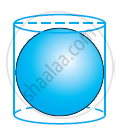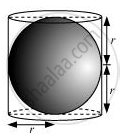# A right circular cylinder just encloses a sphere of radius r (see figure). Find (i) surface area of the sphere, (ii) curved surface area of the cylinder, (iii) ratio of the areas obtained in (i) and (ii). - Mathematics

A right circular cylinder just encloses a sphere of radius r (see figure). Find

(i) surface area of the sphere,

(ii) curved surface area of the cylinder,

(iii) ratio of the areas obtained in (i) and (ii).#### Solution(i) Surface area of sphere = 4πr2

(ii) Height of cylinder = r + r = 2r

CSA of cylinder = 2πrh

= 2π(2r)

= 4πr2

(iii)

"Required ratio "="Surface area of sphere"/"CSA of cylinder"

= (4pir^2)/(4pir^2)= 1/1

Therefore, the ratio between these two surface areas is 1:1.

Concept: Surface Area of a Sphere
Is there an error in this question or solution?

#### APPEARS IN

NCERT Class 9 Maths
Chapter 13 Surface Area and Volumes
Exercise 13.4 | Q 9 | Page 225